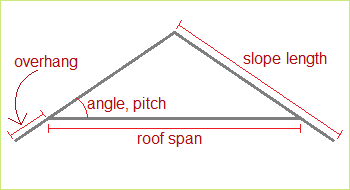# Rafter Length Calculator

 Span ft. in. Pitchor Angle pitch = e.g. 4/12 angle = o Overhang ft. in.

You can use the trigonometry to determine the rafter slope length for an angled roof, all you need to know is the roof span, the pitch or angle, and the length of overhang. Using the calculator on the left or the formula explained below, you can quickly compute the length of the sloped rafter.

The image below shows how all of the roofing measurements and dimensions are interrelated:If the sloped length of the truss rafter is L, the roof span S, the roof angle A, and the overhang V, then the equation for L is

L = V + R/(2cos(A))

The equivalent formula using the pitch, P, is

L = V + (R/2)sqrt(1+P2)

Example 1:

A roof has a span of 14 feet, an angle of 30°, and an over hang of 1 foot. Then the length of the top rafter is

L = 1 + 14/(2cos(30°))
= 1 + 14/1.72305
= 9.0829 feet, or 9' 1"

Example 2:

A roof's span is 21 feet, with 9 inches of overhang, and pitch of 5/12. Here we have R = 21, V = 0.75 (since 9 inches is 3/4 or 0.75 of a foot) and P = 5/12.

L = 0.75 + (21/2)sqrt(1 + (5/12)2)
= 0.75 + (10.5)sqrt(169/144)
= 0.75 + 11.375
= 12.125 feet, or 12' 1" 1/2"